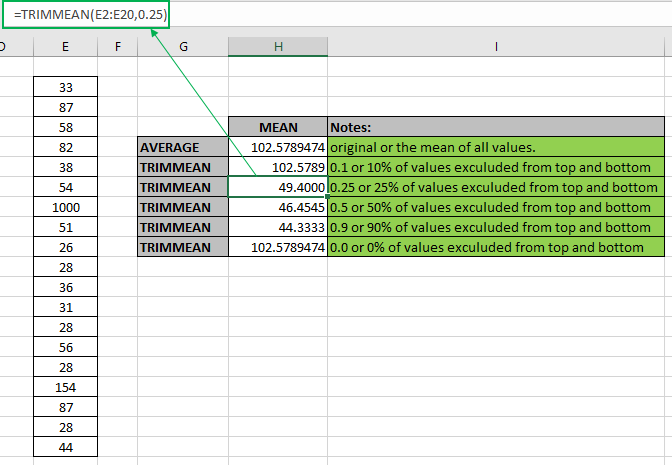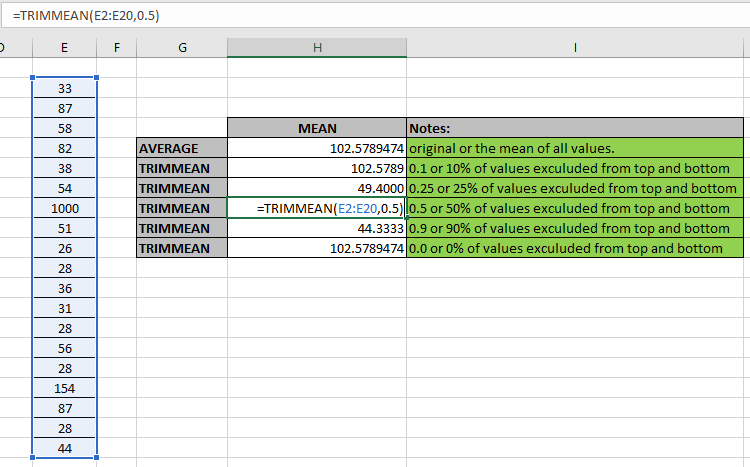# How to use the TRIMMEAN Function in ExcelIn this article, we will learn about how to use the TRIMMEAN function in Excel to calculate the Average or mean for the range of values excluding values by percentage.

 K (in decimal) percentage Exclude values from a sorted array 0 0% none 0.1 10% 10 percent of 30 values = 3 values 0.2 20% 20% percent of 20 values = 4 values 0.3 30% 30% percent of 10 values = 3 values 0.4 40% 40% percent of 10 values = 4 values 0.5 50% 50% percent of 10 values = 5 values 0.6 60% 60% percent of 10 values = 6 values 0.7 70% 70% percent of 10 values = 7 values 0.8 80% 80% percent of 10 values = 8 values 0.9 90% 90% percent of 10 values = 9 values 1 100% Returns #NUM! error

For symmetry, TRIMMEAN excludes a single value from the top and bottom of the sorted data set.

TRIMMEAN function in excel returns a number which is the average or mean of the input numbers and

Syntax of TRIMMEAN function:

=TRIMMEAN (value 1, [value 2], …, k)

value 1 : First value ( necessary )

value 2 : Second value ( optional ). The values can be given using cell reference.

k : percentage in decimal, 0.35 = 35%

Example:

All of these might be confusing to understand. So, let's test this formula via running it on the example shown below. Here we have some random data values to test the TRIMMEAN function in Excel.

Here we have a range of values from E2:E20 cell and we need to get the average or mean for the same range using the below formula

Use the formula:

=TRIMMEAN(E2:E20)See the formula in the cell. Here array value arguments to the function are given using the cell reference method. Using the above formula in the cell to get the average of values or mean of the values.The Average or mean for the random data values considering all number values using TRIMMEAN is 102.5789474 and AVERAGE function returns the same result 102.5789474 for the same data set values. But as we change the value of k, the value decreases from the actual value. This difference occurs as the number of values considered decreases in the formula changes.

If the number of values to be extracted is even then the formula deducts two values from the top and two from the bottom in the data sorted. But If the number of values to be extracted is odd. The it extracts two from top and one from bottom
Here are some observational notes shown below.

Notes:

1. The function takes 255 values as maximum count.
2. If data has some particular criteria, use the AVERAGEIF function & AVERAGEIFS function.
3. The function considers some data set number as date, time, etc. So Careful using the values with date or time values.
4. The value of k >= 0 to K < 1, If k =1 the formula returns #num! error.

Hope this article about How to use the TRIMMEAN function in Excel is explanatory. Find more articles on statistical function formulas here. If you liked our blogs, share it with your fristarts on Facebook . And also you can follow us on Twitter and Facebook . We would love to hear from you, do let us know how we can improve, complement or innovate our work and make it better for you. Write to us at info@exceltip.com.

Related Articles

How to use the AVERAGE function in Excel : AVERAGE function is used in Excel for values considering only numbers which are in number format.

How to use the AVERAGEIF function in Excel : This function takes the average of values in range applying any one condition over the corresponding or the same range.

How to use the AVERAGEIFS function in Excel : This function takes the average of values in range applying any multiple conditions over the corresponding or the same range.

How to Use STDEV Function in Excel : Calculate the standard deviation for the data numbers in excel using the STDEV function.

How to use the VAR function in Excel : Calculate the variance for the sample data numbers in excel using the VAR function.

Popular Articles:

50 Excel Shortcuts to Increase Your Productivity | Get faster at your task. These 50 shortcuts will make you work even faster on Excel.

The VLOOKUP Function in Excel | This is one of the most used and popular functions of excel that is used to lookup value from different ranges and sheets.

COUNTIF in Excel 2016 | Count values with conditions using this amazing function. You don't need to filter your data to count specific values. Countif function is essential to prepare your dashboard.

How to Use SUMIF Function in Excel | This is another dashboard essential function. This helps you sum up values on specific conditions.

Terms and Conditions of use

The applications/code on this site are distributed as is and without warranties or liability. In no event shall the owner of the copyrights, or the authors of the applications/code be liable for any loss of profit, any problems or any damage resulting from the use or evaluation of the applications/code.